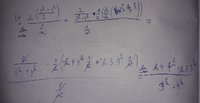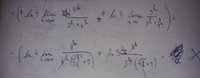# Limit problem

#### Subhotosh Khan

##### Super Moderator
Staff member
•Jomo

#### Ovan

##### New member
View attachment 15967
Please follow the rules of posting in this forum, as enunciated at:

$$\displaystyle \left [\frac{\sqrt[n]{3} + \sqrt[n]{4}}{2} \right ] ^n$$

= $$\displaystyle (4)*\left [\frac{\sqrt[n]{\frac{3}{4}} + 1}{2} \right ] ^n$$

Continue......
I really don't know how to continue.. can u show me some more steps, please?

#### Subhotosh Khan

##### Super Moderator
Staff member
I really don't know how to continue.. can u show me some more steps, please?

What math class are you taking now (or have taken recently) - e.g.- precalculus, advanced algebra, calculus-I, etc.?

Have you studied Taylor's expansion formula?

Have you studied formula for Binomial expansion?

#### Ovan

##### New member

What math class are you taking now (or have taken recently) - e.g.- precalculus, advanced algebra, calculus-I, etc.?

Have you studied Taylor's expansion formula?

Have you studied formula for Binomial expansion?
I have studied both but I don't know how to use it in this example.

#### Subhotosh Khan

##### Super Moderator
Staff member
I have studied both but I don't know how to use it in this example.
Please expand using Binomial expansion theorem (first few terms):

(1 + x)n = ?

#### MarkFL

##### Super Moderator
Staff member
I would state:

$$\displaystyle L=\lim_{n\to\infty}\left(\left(\frac{(3)^{\frac{1}{n}}+(4)^{\frac{1}{n}}}{2}\right)^n\right)$$

Taking the natural log of both sides, we eventually get:

$$\displaystyle \ln(L)=\lim_{n\to\infty}\left(\frac{\ln\left(\dfrac{(3)^{\frac{1}{n}}+(4)^{\frac{1}{n}}}{2}\right)}{\dfrac{1}{n}}\right)$$

Now you have the indeterminate for 0/0 and may apply L'Hôpital's Rule.•Subhotosh Khan

#### Subhotosh Khan

##### Super Moderator
Staff member
I would state:

$$\displaystyle L=\lim_{n\to\infty}\left(\left(\frac{(3)^{\frac{1}{n}}+(4)^{\frac{1}{n}}}{2}\right)^n\right)$$

Taking the natural log of both sides, we eventually get:

$$\displaystyle \ln(L)=\lim_{n\to\infty}\left(\frac{\ln\left(\dfrac{(3)^{\frac{1}{n}}+(4)^{\frac{1}{n}}}{2}\right)}{\dfrac{1}{n}}\right)$$

Now you have the indeterminate for 0/0 and may apply L'Hôpital's Rule.Again assumed things that I should not have assumed. Somehow I thought L'Hospital was not allowed (we had spate of those sadistic problems in near-past!)

•MarkFL

#### Ovan

##### New member
Please expand using Binomial expansion theorem (first few terms):

(1 + x)n = ?
(1 + x)n=
I would state:

$$\displaystyle L=\lim_{n\to\infty}\left(\left(\frac{(3)^{\frac{1}{n}}+(4)^{\frac{1}{n}}}{2}\right)^n\right)$$

Taking the natural log of both sides, we eventually get:

$$\displaystyle \ln(L)=\lim_{n\to\infty}\left(\frac{\ln\left(\dfrac{(3)^{\frac{1}{n}}+(4)^{\frac{1}{n}}}{2}\right)}{\dfrac{1}{n}}\right)$$

Now you have the indeterminate for 0/0 and may apply L'Hôpital's Rule.#### MarkFL

##### Super Moderator
Staff member
I would continue from my previous post as follows:

$$\displaystyle \ln(L)=\lim_{n\to\infty}\left(\frac{\dfrac{1}{(3)^{\frac{1}{n}}+(4)^{\frac{1}{n}}}\left(-n^{-2}\left((3)^{\frac{1}{n}}\ln(3)+(4)^{\frac{1}{n}}\ln(4)\right)\right)}{-n^{-2}}\right)$$

Hence:

$$\displaystyle \ln(L)=\lim_{n\to\infty}\left(\dfrac{(3)^{\frac{1}{n}}\ln(3)+(4)^{\frac{1}{n}}\ln(4)}{(3)^{\frac{1}{n}}+(4)^{\frac{1}{n}}}\right)=\ln\left(2\sqrt{3}\right)$$

And so we conclude:

$$\displaystyle L=2\sqrt{3}$$

•Subhotosh Khan

#### Subhotosh Khan

##### Super Moderator
Staff member
First you should observe that:

$$\displaystyle ln \left[ \dfrac{(3)^{\frac{1}{n}}+(4)^{\frac{1}{n}}}{2}\right] = ln[(3)^{\frac{1}{n}}+(4)^{\frac{1}{n}}] - ln(2)$$

and

$$\displaystyle \frac{d}{dn}\left[ \frac{1}{n}\right ]$$ = - $$\displaystyle \ \frac{1}{n^2}$$

now continue......

•MarkFL

#### Subhotosh Khan

##### Super Moderator
Staff member
Mark took you out of your misery - but please work through the intermediate steps.

#### Cubist

##### Full Member
$$\displaystyle \ln(L)=\lim_{n\to\infty}\left(\frac{\ln\left(\dfrac{(3)^{\frac{1}{n}}+(4)^{\frac{1}{n}}}{2}\right)}{\dfrac{1}{n}}\right)$$

Now you have the indeterminate for 0/0 and may apply L'Hôpital's Rule.Or another idea is performing the change of variable x=1/n to MarkFL's suggestion which makes applying L'Hôpital's rule easy. I ended up with...

$$\displaystyle \ln(L) =\lim_{x\to0}\left( \frac{3^x\cdot \ln\left(3\right)+2\mathinner{\times} 4^x\cdot \ln\left(2\right)}{3^x+4^x} \right)$$

and from there it's simple!

•MarkFL

#### MarkFL

##### Super Moderator
Staff member
Mark took you out of your misery - but please work through the intermediate steps.

It appeared to me that the OP was actually already at the doorstep in the first attached image, having gotten to this point:

$$\displaystyle \ln(L)=\lim_{n\to\infty}\left(\dfrac{(3)^{\frac{1}{n}}\ln(3)+(4)^{\frac{1}{n}}\ln(4)}{(3)^{\frac{1}{n}}+(4)^{\frac{1}{n}}}\right)$$

Now, we only have to recognize this is a determinate form to complete the problem.

$$\displaystyle \ln(L)=\frac{\ln(3)+\ln(4)}{2}=\frac{1}{2}\ln(12)=\ln(\sqrt{12})=\ln(2\sqrt{3})$$

And the conclusion I posted above then follows.•Ovan, Subhotosh Khan and Cubist

#### Ovan

##### New member
Thank you for helping me out .